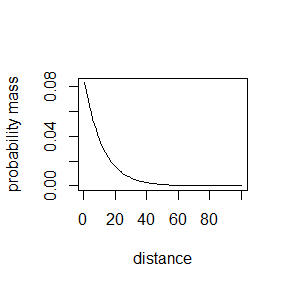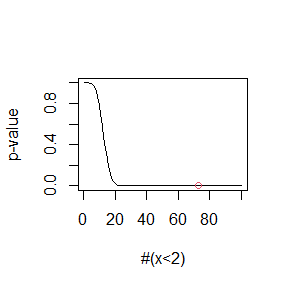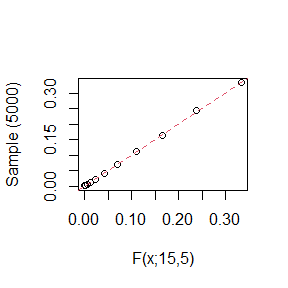# Introduction

This small package contains the Liland distribution for distances between discrete events in fixed time with probability mass, cumulative distribution, quantile function, random number generator, simulation functions and a test for over representation of short distances.

# Example

An example of its use is found in bacterial gene regulation where genes along a chromosome are regulated or not regulated. One may ask if the distances between regulated genes are random or tend to cluster, e.g. as operons. In the following example we have $$R=1949$$ genes (trials) of which $$r=162$$ are regulated (success).

library(fixedTimeEvents)
R <- 1949; r <- 162
Liland(R, r)
##      Mean  Variance
##  11.96319 129.55528

## Probability mass

R <- 1949; r <- 162
dL <- dLiland(1:100, R, r)
plot(dL, type = 'l', xlab = "distance", ylab = "probability mass")## Testing

A test for over representation of short distances can be performed, e.g. for distances shorter than 2 ($$x<2$$).

Lt <- Liland.test(1:100, 1, R, r)
Liland.crit(1, R, r)
##  19
plot(Lt, type='l', xlab='#(x<2)', ylab='p-value')
points(73, Liland.test(73, 1, R, r), col = 2)## Simulated distribution

A comparison between distances obtained from sampling from the Bernoulli distribution with a fixed number of successes and the theoretical values from the Liland distribution follows.

sL <- simLiland(5000, 15,5) # 5000 samples, R = 15, r = 5
qqplot(dLiland(1:length(sL),15,5),sL,
xlab='F(x;15,5)', ylab='Sample (5000)')
abline(0,1, lty=2, col=2)